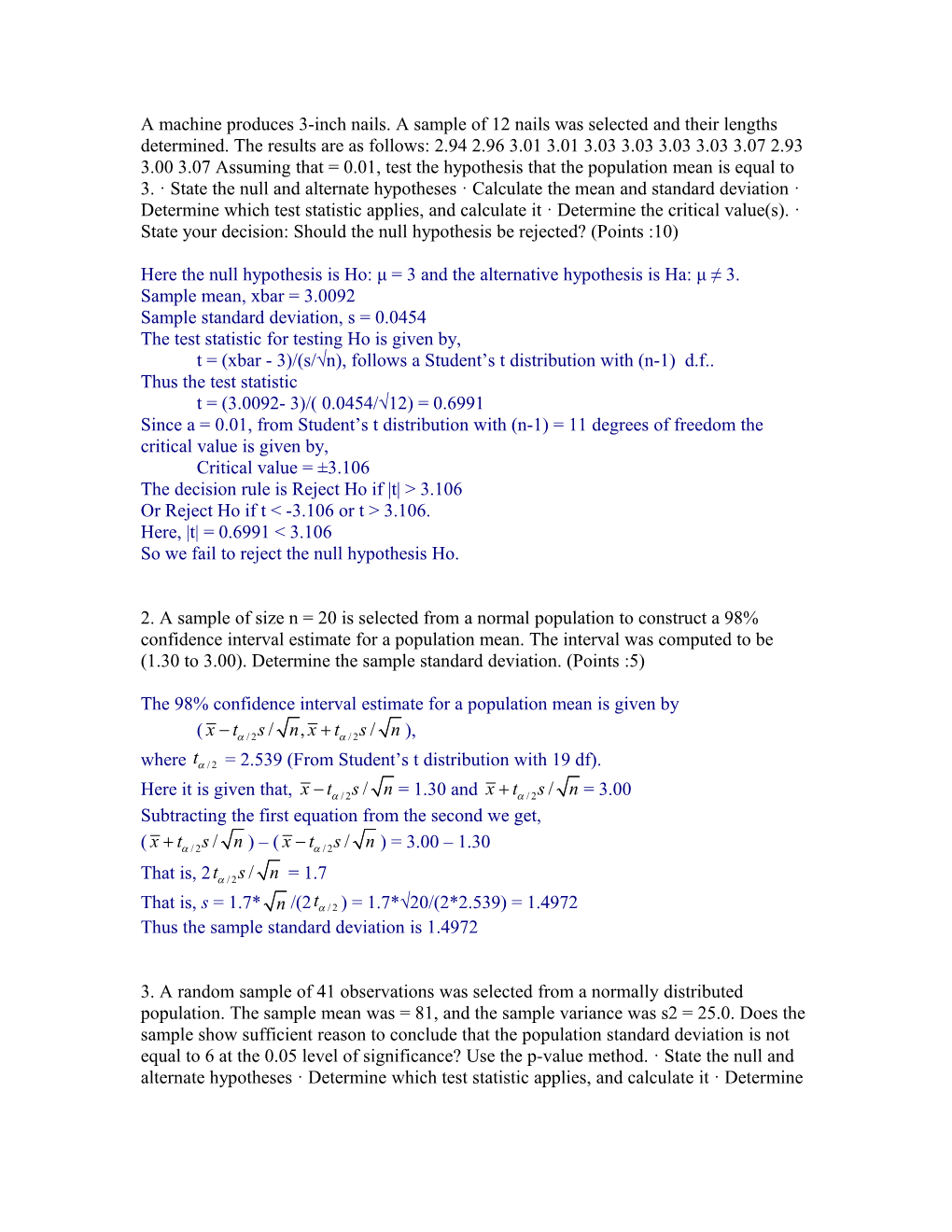# A Machine Produces 3-Inch NailsA machine produces 3-inch nails. A sample of 12 nails was selected and their lengths determined. The results are as follows: 2.94 2.96 3.01 3.01 3.03 3.03 3.03 3.03 3.07 2.93 3.00 3.07 Assuming that = 0.01, test the hypothesis that the population mean is equal to 3. · State the null and alternate hypotheses · Calculate the mean and standard deviation · Determine which test statistic applies, and calculate it · Determine the critical value(s). · State your decision: Should the null hypothesis be rejected? (Points :10)

Here the null hypothesis is Ho: μ = 3 and the alternative hypothesis is Ha: μ ≠ 3.

Sample mean, xbar = 3.0092

Sample standard deviation, s = 0.0454

The test statistic for testing Ho is given by,

t = (xbar - 3)/(s/√n), follows a Student’s t distribution with (n-1) d.f..

Thus the test statistic

t = (3.0092- 3)/(0.0454/√12) = 0.6991

Since a = 0.01, from Student’s t distribution with (n-1) = 11 degrees of freedom the critical value is given by,

Critical value = ±3.106

The decision rule is Reject Ho if |t| > 3.106

Or Reject Ho if t < -3.106 or t > 3.106.

Here, |t| = 0.6991 3.106

So we fail to reject the null hypothesis Ho.

2. A sample of size n = 20 is selected from a normal population to construct a 98% confidence interval estimate for a population mean. The interval was computed to be (1.30 to 3.00). Determine the sample standard deviation. (Points :5)

The 98% confidence interval estimate for a population mean is given by

(),

where = 2.539 (From Student’s t distribution with 19 df).

Here it is given that, = 1.30 and = 3.00

Subtracting the first equation from the second we get,

() – () = 3.00 – 1.30

That is, 2 = 1.7

That is, s = 1.7*/(2) = 1.7*√20/(2*2.539) = 1.4972

Thus the sample standard deviation is 1.4972

3. A random sample of 41 observations was selected from a normally distributed population. The sample mean was = 81, and the sample variance was s2 = 25.0. Does the sample show sufficient reason to conclude that the population standard deviation is not equal to 6 at the 0.05 level of significance? Use the p-value method. · State the null and alternate hypotheses · Determine which test statistic applies, and calculate it · Determine the corresponding probability, and compare to · State your decision: Should the null hypothesis be rejected? (Points :10)

Here the null hypothesis is Ho: σ =6 and the alternative hypothesis is Ho: σ ≠6

The test statistic for testing Ho is given by,

χ2 = (n-1)s2/62, follows a Chi-square distribution with (n-1)= 40 degrees of freedom.

Thus the test statistic

χ2 = (41 -1)(25.0)/62 = 40*25/36 = 27.7778

The p-value of the test is given by

p-value = P[χ2 > 27.7778] = 0.9279

Here it is given that, a = 0.05

Thus, p-value > a

[The p-value is obtained using the Excel formula =CHIDIST(27.7778,40)]

The decision rule is reject Ho if the p-value < 0.05

Here, p-value > 0.05

So we fail to reject the null hypothesis Ho.

Thus the sample does not show sufficient reason to conclude that the population standard deviation is not equal to 6 at the 0.05 level of significance.

4. An insurance company states that 75% of its claims are settled within 5 weeks. A consumer group selected a random sample of 45 of the company’s claims and found 35 of the claims were settled within 5 weeks. Is there enough evidence to support the consumer group’s claim that fewer than 75% of the claims were settled within 5 weeks? Test using the traditional approach with a = 0.05. · State the null and alternate hypotheses · Calculate the sample proportion · Determine which test statistic applies, and calculate it · Determine the critical value(s). · State your decision: Should the null hypothesis be rejected? (Points :10)

Here the null hypothesis is Ho: p = 0.75 and the alternative hypothesis is Ha: p0.75.

The sample proportion, pbar = x/n = 35/45 = 0.7778

The test statistic for testing Ho is given by,

z = (pbar – 0.75)/Sqrt(0.75*0.25/n), follows Standard Normal distribution

Thus the test statistic,

z = (0.7778 – 0.75)/Sqrt(0.75*0.25/45) = 0.4303

Since a = 0.05, the critical value is given by,

Critical value = - 1.645.

The decision rule is Reject Ho if z< -1.645

Here, z = 0.4303 > -1.645

So we fail to reject the null hypothesis Ho.

Thus there is not enough evidence to support the consumer group’s claim that fewer than 75% of the claims were settled within 5 weeks